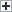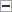Understanding the High-Low MethodWhen it comes to cost accounting, the high-low method is an approach that’s used to break mixed costs into either a variable or fixed cost. Although it’s straightforward, it’s important to do multiple analyses because outlier costs from the available data can sometimes misconstrue operating costs. This calculation occurs by looking at the periods with the most and least activity, as well as the total costs for both the high and low periods.

In order to get results for the high-low method, the variable cost and the fixed cost must be determined first. Once these are established, they are entered into the cost model formula.

Variable Cost is determined as follows:

VC = Highest Activity Cost – Lowest Activity Cost / Highest Activity Units – Lowest Activity Units

The next step is to calculate the Fixed Cost as follows:

FC = Highest Activity Cost – (VC x Highest Activity Units)

Now that the fixed and variable costs are known, the high-low cost can be determined:

High-Low Cost Model = Fixed Cost + (Variable Cost x Unit Activity)

Understanding it Through a Real-World Example

Looking at a furniture manufacturer, it’s good to focus on one product to see how the high-low method works:

The first step is to list production that includes each month, the product produced (let’s say it’s tables), and how much it cost to produce all tables each month. The list could be as follows:

Months Units Produced Total Cost (\$)
January 153 6,650
February 106 5,653
March 120 6,185
April 126 6,120
May 100 4,888
June 133 6,650
July 113 5,852
August 93 4,988
September 153 6,783
October 166 7,382
November 146 6,783
December 160 7,581

The greatest output or activity for the furniture store happened in October when it produced the highest number of tables: 166 at a cost of \$7,382. In August, the furniture store produced the fewest number of tables at 93, manufactured at a cost of \$4,988.

Even though the cost may not be the greatest for the peak and valley of production, the corresponding costs for those respective figures is what will be used.

Now that we’ve identified the relevant data, the first task is to determine the variable cost.

VC = Total Cost of High Activity – Total Cost of Low Activity / Highest Activity Unit – Lowest Activity Unit

VC = \$7,382 – \$4,988 / 166 – 93

VC = \$2,394 / 73 = \$32.80 per table

Then fixed costs must be calculated:

Total Cost = (VC x Units Produced) + Total Fixed Cost

\$7,382 = (\$32.80 x 166) + TFC

\$7,382 = \$5,444.80 + TFC

TFC = \$7,382 – \$5,444.80 = \$1,937.20

It’s important to remember that variable costs are per unit.

Now that we have the total fixed cost, we can then create the total cost equation:

Total Cost = Total Fixed Cost + (VC x Units Produced)

Total Cost = \$1,937.20 + (\$32.80 x 166) = \$7,382

This demonstrates the comprehensive costs for the tables made by the furniture store.

Further Considerations

The high-low method is a quick way to analyze costs. Since it only necessitates the peak and lulls of production data and costs, it can be done more often, along with helping companies plan with limited data to estimate future unit costs.

It’s important to run multiple types of cost analysis because high and low measurements might not give a full picture of costs. Although these two data points may not be the best overall picture of costs a business experiences at those volume levels, it can be an effective measurement until more data becomes available.

Disclaimer